# Is the gas constant the same for all gases

Page - 11 -

II. The gas laws

###Gas equation for an ideal gas

Derivation: In the two equations by Gay-Lussac and Boyle-Mariotte, one variable was always constant, in the first case the pressure and in the second the temperature. However, in order to be able to calculate without restrictions, one needs an equation that describes the relationship between all three variables (pressure, temperature and volume). To do this, one has to summarize the laws of Gay-Lussac and Boyle-Mariotte. A system is assumed that has a temperature T1, a print p1 and a volume V1 Has: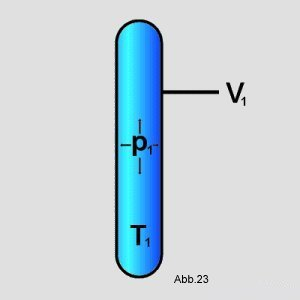The pressure is changed to p2 and the temperature to T2. The question is: How does the volume V look then2 out?

In the first step the pressure of p1 on p2 changed. The temperature is kept the same. Hence Boyle-Mariotte's law can be applied. To do this, use formula (10) and solve it for Vx on (In the graphic example, the pressure elevated):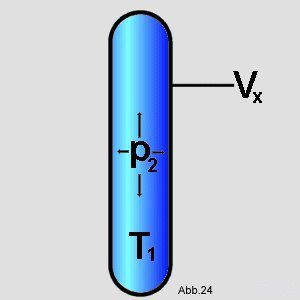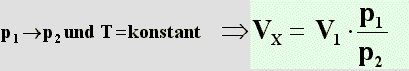(11)

The new pressure p is then maintained2 constant and only changes the temperature of T1 on T2. This process can be calculated using Gay-Lussac's law. Formula (7) is used for this purpose (in the graphic example, the temperature is elevated):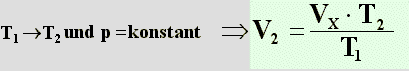(12)

Put V into the second equationx from the first equation, and get the following: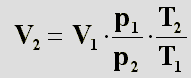(13)

When converted, the result is:(14)

 It also follows from this that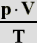is constant.

We now know that the product of volume and pressure in relation to temperature has a constant value. In order to be able to calculate this, you need the three connected values ​​at a certain point. The reference state can be selected as that of an ideal gas at a pressure of 1.013 bar and a temperature of 273.15 K (= 0 C). At this point, according to Avogadro, the volume of one mole of an ideal gas is 22.414 l (= 22.414 dm3). Therefore you have to include the amount of substance n in order to get the molar ratios. These values ​​are provided with 0 as an index, as they refer to the point at 0 C.
You can now plug them into the equation and get:(15)

The number highlighted in green and the units behind it are always the same, no matter which point you looked at. They are the constant that is called R and is called "General Gas Constant". Now you can ideal gas law formulate:(16)

Example:
2 mol of oxygen O are allowed2 bubble into an inverted vessel filled with water:Given: The outside temperature is 25 C and the air pressure is 1.013 bar.

Question: What is the volume that the oxygen will take up?

Solution: The ideal gas law can be applied here. It reads as follows:The amount of substance n is 2 mol, the temperature is 298K (25 C + 273) and the pressure is 1.013 bar (normal air pressure). You also need the value of the general gas constant R. It is: 0.083 (cm3 * bar) / K (see formula (15)). These values ​​are now inserted into the formula:This is now solved for the volume V:Answer: The result is an oxygen volume of 48.83 dm3 or liter (1 dm3 = 1 liter).